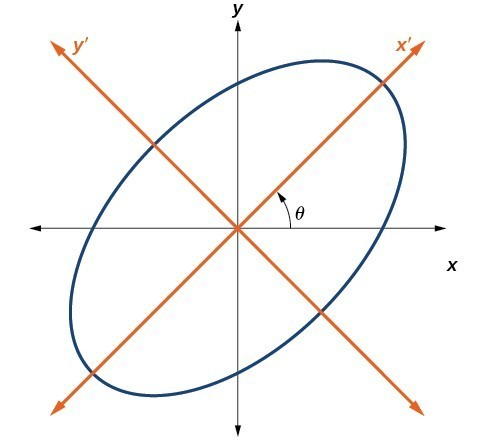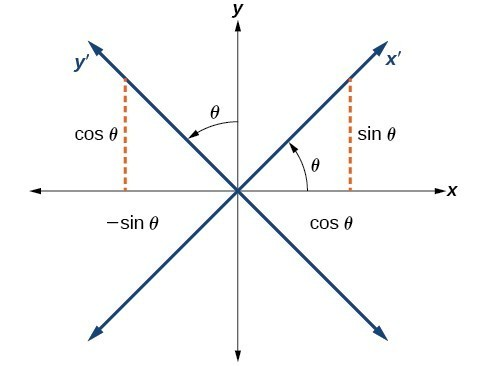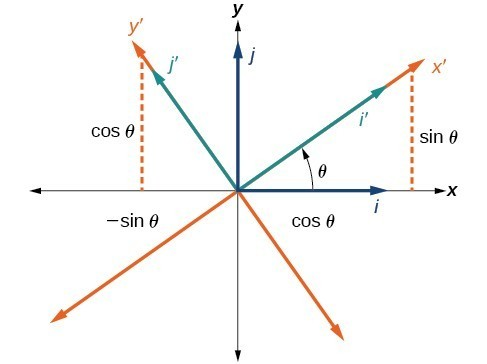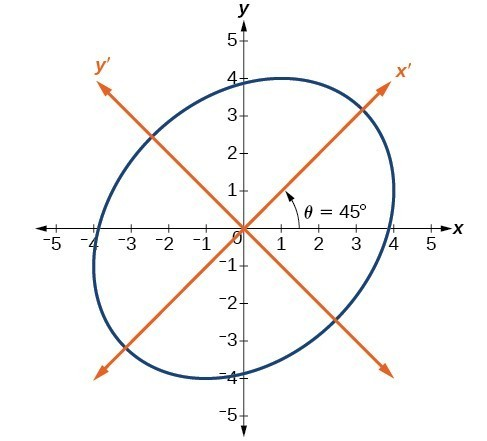## Finding a New Representation of the Given Equation after Rotating through a Given Angle

Until now, we have looked at equations of conic sections without an $xy$ term, which aligns the graphs with the x– and y-axes. When we add an $xy$ term, we are rotating the conic about the origin. If the x– and y-axes are rotated through an angle, say $\theta$, then every point on the plane may be thought of as having two representations: $\left(x,y\right)$ on the Cartesian plane with the original x-axis and y-axis, and $\left({x}^{\prime },{y}^{\prime }\right)$ on the new plane defined by the new, rotated axes, called the x’-axis and y’-axis.Figure 3. The graph of the rotated ellipse ${x}^{2}+{y}^{2}-xy – 15=0$

We will find the relationships between $x$ and $y$ on the Cartesian plane with ${x}^{\prime }$ and ${y}^{\prime }$ on the new rotated plane.Figure 4. The Cartesian plane with x- and y-axes and the resulting x′− and y′−axes formed by a rotation by an angle $\text{ }\theta$.

The original coordinate x– and y-axes have unit vectors $i$ and $j$. The rotated coordinate axes have unit vectors ${i}^{\prime }$ and ${j}^{\prime }$. The angle $\theta$ is known as the angle of rotation. We may write the new unit vectors in terms of the original ones.

$\begin{array}{l}{i}^{\prime }=\cos \text{ }\theta i+\sin \text{ }\theta j\hfill \\ {j}^{\prime }=-\sin \text{ }\theta i+\cos \text{ }\theta j\hfill \end{array}$Figure 5. Relationship between the old and new coordinate planes.

Consider a vector $u$ in the new coordinate plane. It may be represented in terms of its coordinate axes.

$\begin{array}{ll}u={x}^{\prime }{i}^{\prime }+{y}^{\prime }{j}^{\prime }\hfill & \hfill \\ u={x}^{\prime }\left(i\text{ }\cos \text{ }\theta +j\text{ }\sin \text{ }\theta \right)+{y}^{\prime }\left(-i\text{ }\sin \text{ }\theta +j\text{ }\cos \text{ }\theta \right)\hfill & \begin{array}{cccc}& & & \end{array}\text{Substitute}.\hfill \\ u=ix\text{‘}\text{ }\cos \text{ }\theta +jx\text{‘}\text{ }\sin \text{ }\theta -iy\text{‘}\text{ }\sin \text{ }\theta +jy\text{‘}\text{ }\cos \text{ }\theta \hfill & \begin{array}{cccc}& & & \end{array}\text{Distribute}.\hfill \\ u=ix\text{‘}\text{ }\cos \text{ }\theta -iy\text{‘}\text{ }\sin \text{ }\theta +jx\text{‘}\text{ }\sin \text{ }\theta +jy\text{‘}\text{ }\cos \text{ }\theta \hfill & \begin{array}{cccc}& & & \end{array}\text{Apply commutative property}.\hfill \\ u=\left(x\text{‘}\text{ }\cos \text{ }\theta -y\text{‘}\text{ }\sin \text{ }\theta \right)i+\left(x\text{‘}\text{ }\sin \text{ }\theta +y\text{‘}\text{ }\cos \text{ }\theta \right)j\hfill & \begin{array}{cccc}& & & \end{array}\text{Factor by grouping}.\hfill \end{array}$

Because $u={x}^{\prime }{i}^{\prime }+{y}^{\prime }{j}^{\prime }$, we have representations of $x$ and $y$ in terms of the new coordinate system.

$\begin{array}{c}x={x}^{\prime }\cos \text{ }\theta -{y}^{\prime }\sin \text{ }\theta \\ \text{and}\\ y={x}^{\prime }\sin \text{ }\theta +{y}^{\prime }\cos \text{ }\theta \end{array}$

### A General Note: Equations of Rotation

If a point $\left(x,y\right)$ on the Cartesian plane is represented on a new coordinate plane where the axes of rotation are formed by rotating an angle $\theta$ from the positive x-axis, then the coordinates of the point with respect to the new axes are $\left({x}^{\prime },{y}^{\prime }\right)$. We can use the following equations of rotation to define the relationship between $\left(x,y\right)$ and $\left({x}^{\prime },{y}^{\prime }\right):$

$x={x}^{\prime }\cos \text{ }\theta -{y}^{\prime }\sin \text{ }\theta$

and

$y={x}^{\prime }\sin \text{ }\theta +{y}^{\prime }\cos \text{ }\theta$

### How To: Given the equation of a conic, find a new representation after rotating through an angle.

1. Find $x$ and $y$ where $x={x}^{\prime }\cos \text{ }\theta -{y}^{\prime }\sin \text{ }\theta$ and $y={x}^{\prime }\sin \text{ }\theta +{y}^{\prime }\cos \text{ }\theta$.
2. Substitute the expression for $x$ and $y$ into in the given equation, then simplify.
3. Write the equations with ${x}^{\prime }$ and ${y}^{\prime }$ in standard form.

### Example 2: Finding a New Representation of an Equation after Rotating through a Given Angle

Find a new representation of the equation $2{x}^{2}-xy+2{y}^{2}-30=0$ after rotating through an angle of $\theta =45^\circ$.

### Solution

Find $x$ and $y$, where $x={x}^{\prime }\cos \text{ }\theta -{y}^{\prime }\sin \text{ }\theta$ and $y={x}^{\prime }\sin \text{ }\theta +{y}^{\prime }\cos \text{ }\theta$.

Because $\theta =45^\circ$,

$\begin{array}{l}\hfill \\ x={x}^{\prime }\cos \left(45^\circ \right)-{y}^{\prime }\sin \left(45^\circ \right)\hfill \\ x={x}^{\prime }\left(\frac{1}{\sqrt{2}}\right)-{y}^{\prime }\left(\frac{1}{\sqrt{2}}\right)\hfill \\ x=\frac{{x}^{\prime }-{y}^{\prime }}{\sqrt{2}}\hfill \end{array}$

and

$\begin{array}{l}\\ \begin{array}{l}y={x}^{\prime }\sin \left(45^\circ \right)+{y}^{\prime }\cos \left(45^\circ \right)\hfill \\ y={x}^{\prime }\left(\frac{1}{\sqrt{2}}\right)+{y}^{\prime }\left(\frac{1}{\sqrt{2}}\right)\hfill \\ y=\frac{{x}^{\prime }+{y}^{\prime }}{\sqrt{2}}\hfill \end{array}\end{array}$

Substitute $x={x}^{\prime }\cos \theta -{y}^{\prime }\sin \theta$ and $y={x}^{\prime }\sin \text{ }\theta +{y}^{\prime }\cos \text{ }\theta$ into $2{x}^{2}-xy+2{y}^{2}-30=0$.

$2{\left(\frac{{x}^{\prime }-{y}^{\prime }}{\sqrt{2}}\right)}^{2}-\left(\frac{{x}^{\prime }-{y}^{\prime }}{\sqrt{2}}\right)\left(\frac{{x}^{\prime }+{y}^{\prime }}{\sqrt{2}}\right)+2{\left(\frac{{x}^{\prime }+{y}^{\prime }}{\sqrt{2}}\right)}^{2}-30=0$

Simplify.

$\begin{array}{ll}\overline{)2}\frac{\left({x}^{\prime }-{y}^{\prime }\right)\left({x}^{\prime }-{y}^{\prime }\right)}{\overline{)2}}-\frac{\left({x}^{\prime }-{y}^{\prime }\right)\left({x}^{\prime }+{y}^{\prime }\right)}{2}+\overline{)2}\frac{\left({x}^{\prime }+{y}^{\prime }\right)\left({x}^{\prime }+{y}^{\prime }\right)}{\overline{)2}}-30=0\hfill & \begin{array}{cccc}& & & \end{array}\text{FOIL method}\hfill \\ \text{ }{x}^{\prime }{}^{2}{\overline{)-2{x}^{\prime }y}}^{\prime }+{y}^{\prime }{}^{2}-\frac{\left({x}^{\prime }{}^{2}-{y}^{\prime }{}^{2}\right)}{2}+{x}^{\prime }{}^{2}\overline{)+2{x}^{\prime }{y}^{\prime }}+{y}^{\prime }{}^{2}-30=0\hfill & \begin{array}{cccc}& & & \end{array}\text{Combine like terms}.\hfill \\ \text{ }2{x}^{\prime }{}^{2}+2{y}^{\prime }{}^{2}-\frac{\left({x}^{\prime }{}^{2}-{y}^{\prime }{}^{2}\right)}{2}=30\hfill & \begin{array}{cccc}& & & \end{array}\text{Combine like terms}.\hfill \\ \text{ }2\left(2{x}^{\prime }{}^{2}+2{y}^{\prime }{}^{2}-\frac{\left({x}^{\prime }{}^{2}-{y}^{\prime }{}^{2}\right)}{2}\right)=2\left(30\right)\hfill & \begin{array}{cccc}& & & \end{array}\text{Multiply both sides by 2}.\hfill \\ \text{ }4{x}^{\prime }{}^{2}+4{y}^{\prime }{}^{2}-\left({x}^{\prime }{}^{2}-{y}^{\prime }{}^{2}\right)=60\hfill & \begin{array}{cccc}& & & \end{array}\text{Simplify}.\hfill \\ \text{ }4{x}^{\prime }{}^{2}+4{y}^{\prime }{}^{2}-{x}^{\prime }{}^{2}+{y}^{\prime }{}^{2}=60\hfill & \begin{array}{cccc}& & & \end{array}\text{Distribute}.\hfill \\ \text{ }\frac{3{x}^{\prime }{}^{2}}{60}+\frac{5{y}^{\prime }{}^{2}}{60}=\frac{60}{60}\hfill & \begin{array}{cccc}& & & \end{array}\text{Set equal to 1}.\hfill \end{array}$

Write the equations with ${x}^{\prime }$ and ${y}^{\prime }$ in the standard form.

$\frac{{{x}^{\prime }}^{2}}{20}+\frac{{{y}^{\prime }}^{2}}{12}=1$

This equation is an ellipse. Figure 6 shows the graph.Figure 6